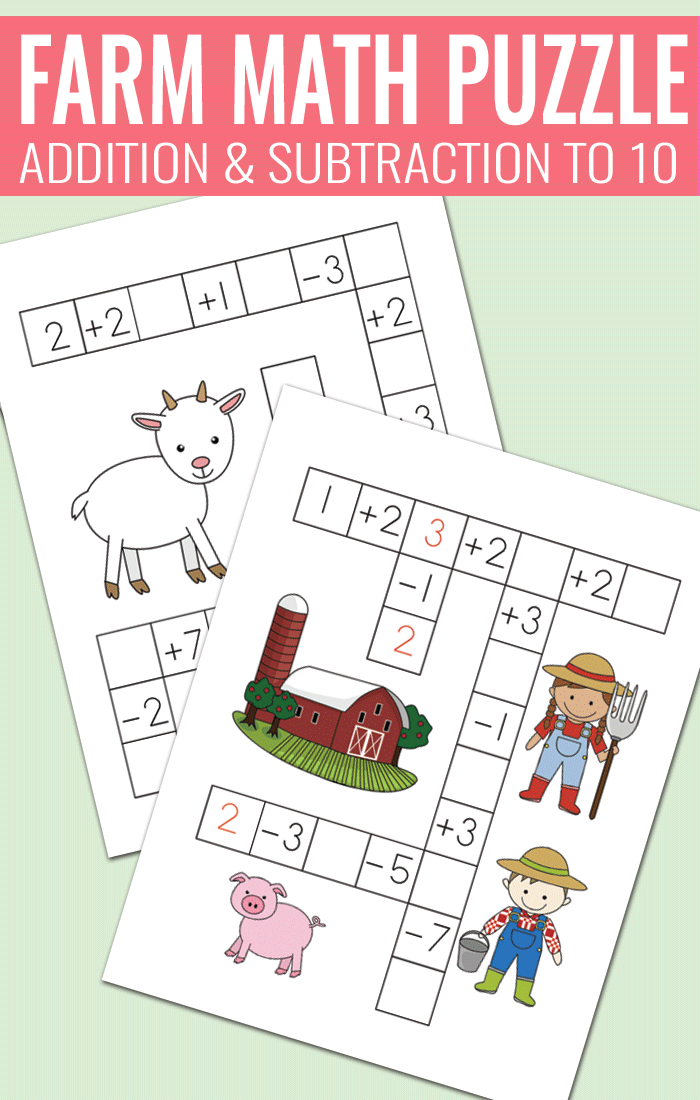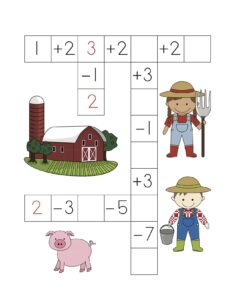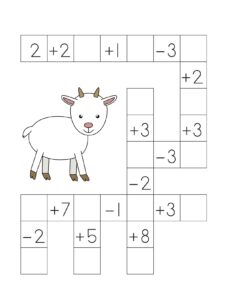# Farm Math Puzzles – Addition and Subtraction Worksheets

Practice addition and subtraction to 10 with these unique farm themed math puzzles. These are perfect for kids in kindergarten and first grade.

This printable resource is a freebie.## FREE Farm Math Puzzles

This easy set of math worksheets is focused on addition and subtraction up to 10.

Students have to solve the crossword puzzle by solving the easy equations and writing down the answers into empty boxes.

Page 1Page 2The first number is given in the upper right corner (ie number 2).

Students look at the number next to that one (ie +2) and solve the equation (ie 2+2).

They write down the solution into the empty box (ie 4). Kids then look at the next number (ie +1) and add or subtract that number from the number they got from the previous equation (ie 4+1).

Students again write down the solution in the empty box (ie 5). They continue until the whole puzzle is solved.

Grab this FREE set of  Addition and Subtraction Puzzle Worksheets

### More Crossword Puzzles From Shop: